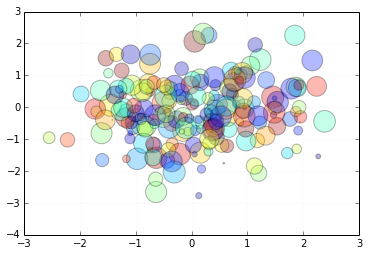# Demo of mpld3¶

This notebook gives a brief overview of mpld3, a package which allows seamless visualization of matplotlib plots using HTML, Javascript, and the D3js package.

One of the nicest parts of the IPython notebook is the ability to embed figures inline with code. For example, we can quickly create a scatter plot as so:

In :
%matplotlib inline
import matplotlib.pyplot as plt
import numpy as np

In :
# Scatter points
fig, ax = plt.subplots()
np.random.seed(0)
x, y = np.random.normal(size=(2, 200))
color, size = np.random.random((2, 200))

ax.scatter(x, y, c=color, s=500 * size, alpha=0.3)
ax.grid(color='lightgray', alpha=0.7)A weakness quickly becomes clear, however: the embedded figure is a simple static PNG image.

This is where mpld3 comes in. Using the simple mpld3.display() command, we can create a fully interactive visualization of the same plot:

In :
import mpld3
mpld3.display(fig)

Out:

Notice that as you hover over the plot, a toolbar appears in the lower left. This has tools to enable panning and zooming, and a button to reset the view once you've explored the plot.

If you'd like to use mpld3 by default for every figure you generate, you can call the following command:

In :
mpld3.enable_notebook()


## Other Plot Types¶

Nearly any type of plot matplotlib can do, mpld3 can visualize as well. Below are some examples:

### A Histogram¶

In :
# Histogram with modified axes/grid
fig = plt.figure()

ax = fig.add_subplot(111, axisbg='#EEEEEE')
ax.grid(color='white', linestyle='solid')

x = np.random.normal(size=1000)
ax.hist(x, 30, histtype='stepfilled', fc='lightblue', alpha=0.5);


### Line Plots with Legend¶

In :
# Draw lines
fig, ax = plt.subplots()
x = np.linspace(-5, 15, 1000)
for offset in np.linspace(0, 3, 4):
ax.plot(x, 0.9 * np.sin(x - offset), lw=5, alpha=0.4,
label="Offset: {0}".format(offset))
ax.set_xlim(0, 10)
ax.set_ylim(-1.2, 1.0)
ax.text(5, -1.1, "Here are some curves", size=18, ha='center')
ax.grid(color='lightgray', alpha=0.7)
ax.legend()

Out:
<matplotlib.legend.Legend at 0x106306450>

### Multiple Subplots with linked axes¶

In :
# multiple subplots, shared axes
fig, ax = plt.subplots(2, 2, figsize=(8, 6),sharex='col', sharey='row')

np.random.seed(0)

for axi in ax.flat:
color = np.random.random(3)
axi.plot(np.random.random(30), lw=2, c=color)
axi.set_title("RGB = ({0:.2f}, {1:.2f}, {2:.2f})".format(*color),
size=14)
axi.grid(color='lightgray', alpha=0.7)


## Plugins¶

One of the key features of mpld3 is the plugin framework. Plugins are a way to specify additional interactivity for your plots. A number of plugins are built-in, and it is also possible to define new, custom plugins for nearly limitless interactive behaviors. For example, here is the built-in Linked Brushing plugin that allows exploration of multi-dimensional datasets:

In :
from mpld3 import plugins

fig, ax = plt.subplots(3, 3, figsize=(6, 6))
ax = ax[::-1]

X = np.random.normal(size=(3, 100))
for i in range(3):
for j in range(3):
ax[i, j].xaxis.set_major_formatter(plt.NullFormatter())
ax[i, j].yaxis.set_major_formatter(plt.NullFormatter())
points = ax[i, j].scatter(X[j], X[i])